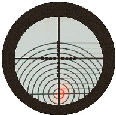The Kitchen Table GunsmithMain menuLaser Bore Sight Calculator by Roy Seifert Click here to purchase a CD with this and all Kitchen Table Gunsmith Articles. Disclaimer:  This article is for entertainment only and is not to be used in lieu of a qualified gunsmith.  Please defer all firearms work to a qualified gunsmith.  Any loads mentioned in this article are my loads for my guns and have been carefully worked up using established guidelines and special tools.  The author assumes no responsibility or liability for use of these loads, or use or misuse of this article.  Please note that I am not a professional gunsmith, just a shooting enthusiast and hobbyist, as well as a tinkerer.  This article explains work that I performed to my guns without the assistance of a qualified gunsmith.  Some procedures described in this article require special tools and cannot/should not be performed without them. Warning:  Disassembling and tinkering with your firearm may void the warranty.  I claim no responsibility for use or misuse of this article.  Again, this article is for entertainment purposes only! Tools and firearms are the trademark/service mark or registered trademark of their respective manufacturers. Introduction I have a laser bore sight that I use to bore sight a rifle after attaching a scope.  The theory is that by bore sighting the rifle you can at least get the shots on paper at 100 yards.  From that point, you can then go to the range and perform the final sight adjustments with your particular loads. The instructions that came with my laser bore sight stated that at twenty-five (25) feet you should adjust the scope for two inches above the laser dot.  This seemed too general to me and I couldn’t see how that would fit for all loads.  Therefore, I developed a laser bore sight calculator that should get me much closer to zero at 100 yards.  The objective here is to determine how far above the red laser dot I should adjust a scope for a 100 yard zero when bore sighting a rifle at 25 feet. There were two pieces of information I needed to know before performing these calculations.  First, I needed to measure the height of my scope above the center line of the bore.  This was easy to obtain just by measuring with a ruler.  Secondly, I must know the departure angle of the bullet.  I can get this from my  ballistic software.  However, I must know the performance of my load and enter that data into the software.  At a minimum, I need to know the muzzle velocity (MV) of the bullet, and it’s ballistic coefficient (BC).  Most bullet manufacturers publish the BC for each of their bullets, and a reloading manual can give me an estimated velocity.  This is going to be close enough to get the bullet on paper at 100 yards. Theory and Geometry If you find this technical explanation too complicated, you can skip it.  You can download my Laser Bore Sight Calculator, an Excel spreadsheet, that will do all the calculations for you.  Just enter the measurement of the distance between the center of the bore and center of the scope, and the departure angle, and the spreadsheet will calculate the distance above the red dot for which you need to adjust your scope at 25 feet.  I had to think about this for a bit and visualize it in my mind.  What I couldn’t get through my head was that the bullet path had nothing to do with bore sighting.  The flight of a bullet is a parabolic curve, but bore sighting involves straight lines.  So I drew a diagram with straight lines so I could visualize what was happening during the bore sighting process.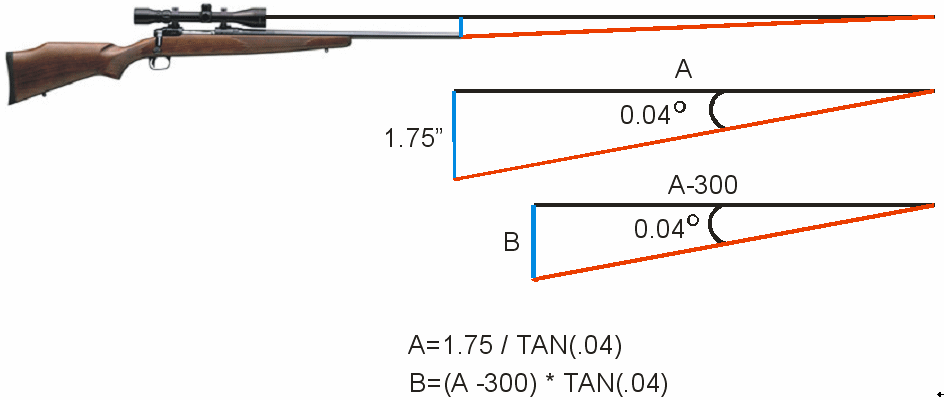When you shoot a rifle, if the rifle was perfectly level with the ground, gravity starts to pull the bullet down immediately, so the bullet would hit low at 100 yards.  Therefore, to hit the center of the target at 100 yards, or any distance for that matter, the rifle has to have a small upward angle.  This angle, called departure angle, and the height of the scope above the bore are the numbers I needed to know. Going back to high school algebra and geometry, by knowing the values of any two parts of a right triangle, I can find the value of any other part.  If you look at the above diagram, the line extending from the scope (line of sight) becomes the base of the triangle.  Because the rifle is at an angle, the line extending from the bore becomes the hypotenuse, and the distance from the center of the bore to the middle of the scope becomes the vertical leg.  The angle where the two lines come together is the departure angle.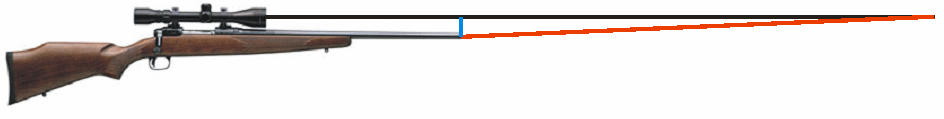Because the scope was level, but the barrel was angled, somewhere out in the distance those two lines would come together.  I needed to find out how far from the bore those two lines intersected.  Once I found that distance, I could subtract 25 feet (300 inches) and find the new value of the leg.  Because the barrel is at an angle, as we move farther from the bore the closer together the two lines become.  So, to properly set up my scope, I need to know how far above the red dot the scope must be adjusted for at 25 feet so the rifle can be zeroed at 100 yards (or at least close). The black lines in the above diagram represent the line of sight from the scope.  The red lines represent the laser projecting from the bore, and the blue lines represent the height of the sight above the bore.  You can also think of the blue lines as the height of the scope cross hairs above the laser dot.  Please note that the triangles in the diagram are not drawn to scale, but the values are accurate. Finding the Angle Finding the angle of the rifle was easy with my ballistic software.  I use Ballistic v4.13 Copyright 1988-1995 by William R. Frenchu.  You can download a free trial copy from Steve’s Reloading Pages.  Other ballistic software packages may also provide the angle of departure, but I only have experience with this software. I used my Remington 700 BDL in 6mm Remington caliber for the calculations.  This rifle is already sighted-in at 100 yards so I can verify my calculations.  Ballistic v4.13 stated that for my load the angle of departure was 0.040 degrees, so that is what I used for my calculations.  I used an estimated muzzle velocity, not actual chronograph values.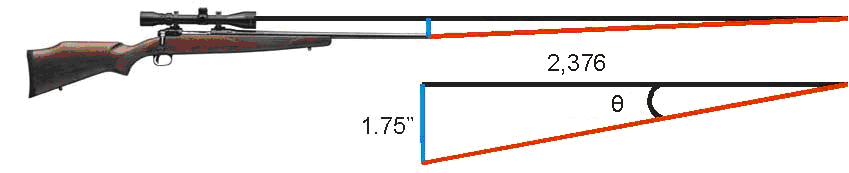Ok, so you don’t have Mr. Frenchu’s excellent program.  You can calculate the angle of departure using the following formula (also available on my Laser Bore Sight Calculator): Angle of departure = ARCTAN(sight height above bore/distance to cross the line of sight) Sight height above the bore is measured from the center of the bore to the top of the front sight, or center of the scope if installed.  The distance to cross the line of sight has to be determined with a ballistic program.  I used Sierra Infinity V6 and using the same 6mm Remington data the bullet crossed the line of sight at 66 yards, which is 2,376 inches.  My scope sits 1.75 inches above the bore so the formula looked like this: ARCTAN(1.75/2,376) = 0.042 degrees ARCTAN is the inverse tangent function.  Looking at the above figure and knowing that the tangent of an angle is the opposite side divided by the adjacent side gave the above formula. Finding the Length of the Base Leg Remember I said that by knowing the values of any two parts of a right triangle, I can find any other value.  I knew the rifle was angled at 0.04 degrees, and the middle of the scope was 1.75” above the center of the bore.  If you look at the second triangle you can see those measurements.  Do you remember SOH-CAH-TOA (rhymes with Crakatoa) from your geometry days?  SOH-CAH-TOA is a memory mnemonic for calculating parts of a right triangle.  It stands for: SOH:  SIN(θ) = opposite over hypotenuse CAH:  COSINE(θ) = adjacent over hypotenuse TOA:  TANGENT(θ) = opposite over adjacent These formulae show the relationships of the lines of the triangle with respect to any one of the angles (θ).  The hypotenuse is always the angled line; the red line in our triangles.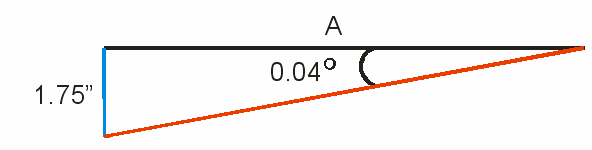In the second triangle, I knew the angle was 0.04 degrees, and I knew the blue line opposite that angle was 1.75 inches.  I needed to find the length of the black line that was adjacent to the angle.  The black line is not the hypotenuse because it is not angled.  I knew opposite and wanted to find adjacent, so I needed the TANGENT function.  The tangent of an angle is the length of the opposite leg divided by the length of the adjacent leg.  Substituting values in the TANGENT formula I got: TANGENT(.04) = 1.75 / A Using some basic algebra to tell me how to find the value of A, first I multiplied both sides by A, which gave me A * TANGENT(.04) = 1.75, then I divided both sides by TANGENT(.04) which gave me A = 1.75 / TANGENT(.04) So, to find the length of line A, the formula was:  1.75 / TANGENT(.04) which was 2506 inches or almost 70 yards.  (Remember, this has nothing to do with bullet flight, this 70 yards is the distance where the line of sight from the scope would touch the line from the laser.) Finding the Height Above the Laser Dot Ok, so now I knew the distance from the bore to where the two lines intersected.  However, because my rifle was 25 feet away from my measuring point (in my case it was a wall inside my home), not 70 yards away, I needed to find out how far above the red dot I should adjust my scope.I started by subtracting 300 inches (25 feet) from the value of the base leg in the second triangle.  That gave me 2206 inches.  Since I now knew the angle (0.04 degrees), and I knew the base leg (2206) I again used the TANGENT formula to find the height of the blue leg (B). TANGENT(.04) = B / 2206 Again, using algebra to solve for B, first I multiplied both sides by 2206 giving me 2206 * TANGENT(.04) = B, so the final formula was: B = 2206 * TANGENT(.04) which gave me an answer of 1.54 inches.  So, at 25 feet, the cross hairs of the scope should be 1 1/2 inches above the red dot. I used the scientific view of the calculator that came with my PC software to perform these calculations.  Again, you can download an Excel spreadsheet that will automatically do the same thing. I tried this formula with my .308 M1A which is also already sighted in for 100 yards.  The scope was 2.5 inches above the bore, and my ballistic software showed the departure angle as 0.037 degrees.  Using my calculator, I got 2.3 inches, which should be where I need to adjust my scope above the red dot at 25 feet.  As you can see the sight height, bullet and caliber make a difference.  And, the bore sight manufacturer’s general measurement of 2 inches high at 25 feet would probably be alright for .30 caliber ammunition.Testing the Theory I created a target with 1/4” rings.  I set up my Remington 700 rifle with the bore sight installed and positioned the target at 25 feet with the laser dot on the center of the target.  The scope reticle was centered at 1 3/4”; my estimate was 1 1/2”.  This variation could be caused by many factors, but most likely was caused by me entering an estimated MV instead of actual MV into my ballistic software.  However, the variation of 1/4” at 25 feet represents 3 inches at 100 yards; good enough to be on the paper.  After all, the point of bore-sighting is to get on the paper, then use live fire to finish sighting in.  So, with some basic geometry and estimated bullet performance I can bore sight my rifle and get it to shoot on the paper.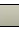© Copyright 2008-2013 Roy Seifert.